### Verification against the Exact Analytical Solution

The generally accepted criterion used to determine the validity of a numerical solution is its coincidence with the known exact analytical solution, and Frost 3D was verified against the analytical solution for the soil freezing problem .

Problem Statement

The 30-day temperature distribution on a section of frozen ground is calculated for the following conditions:
• a +5 oС initial soil temperature;
• a constant -10 oС is maintained on the upper surface of the soil;
• zero heat flow at the lateral and bottom surfaces of the soil section due to insulation.

Table 1: Thermophysical Properties of Ground

 The Number of Computation Soil Thermal Conductivity, W/(m×°С) Volumetric Heat Capacity of Soil, kJ/(m3×°С) Frost Depth, m T ≥ 0 oС T < 0 oС T ≥ 0 oС T < 0 oС Numerical Computation Analytical Solution 1 0.64 0.7 1600 1470 0.2000 0.2011 2 0.64 0.7 3780 2730 0.1875 0.1879 3 2.73 2.9 1600 1470 0.4125 0.4140 4 2.73 2.9 3780 2730 0.3800 0.3816

The dependence of thermophysical properties on temperature (see Table 1) in compliance with the SNiP 2.02.04-88 Norms “Bases and Foundations on Permafrost” is typical for sand, loamy sand and sandy loam with plasticity index Ip < 0.02.

The comparison (Figure 1–4) of the derived numerical and the exact analytical solution for the ground freezing problem revealed a 0.5% error in the frost depth calculation using Frost 3D, and we observe that mesh refinement makes the error approach zero.

Comparison of the solutions obtained in Frost 3D and the exact analytical solution:Figure 1: Results of 1st numerical experiment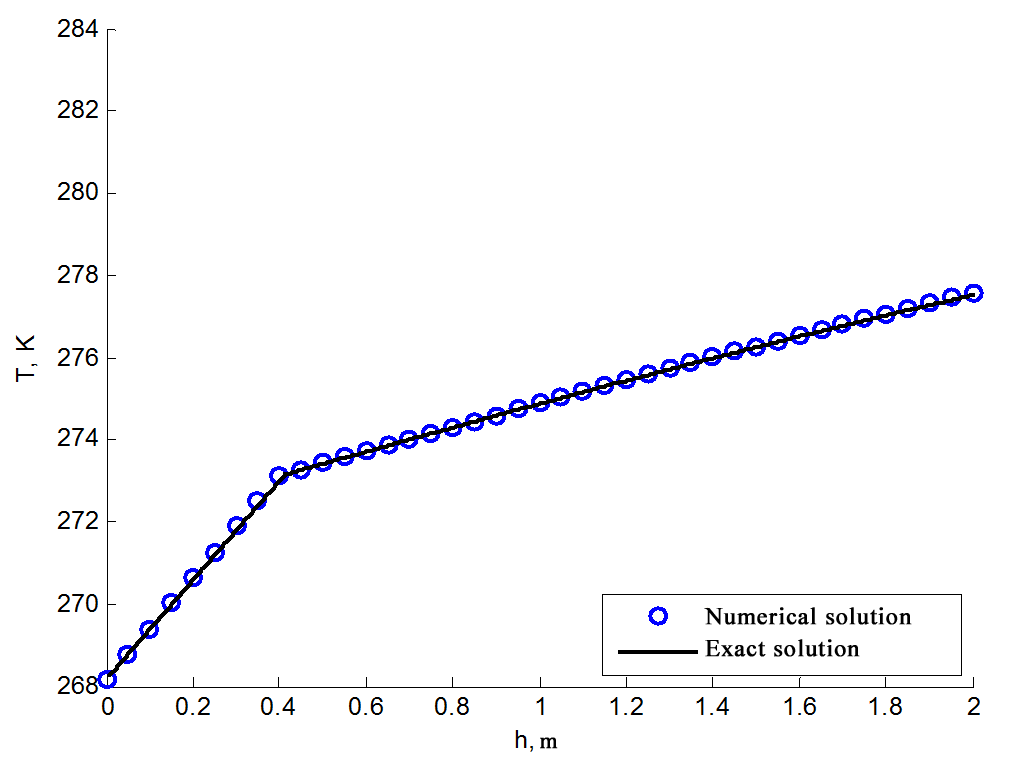Figure 3: Results of 3rd numerical experiment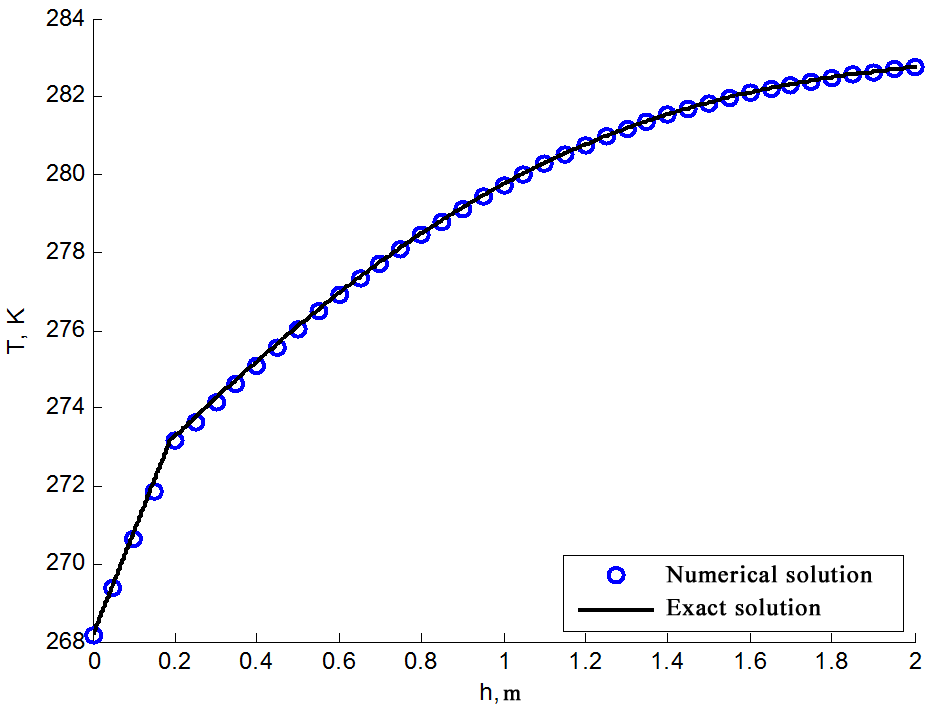Figure 2: Results of 2nd numerical experiment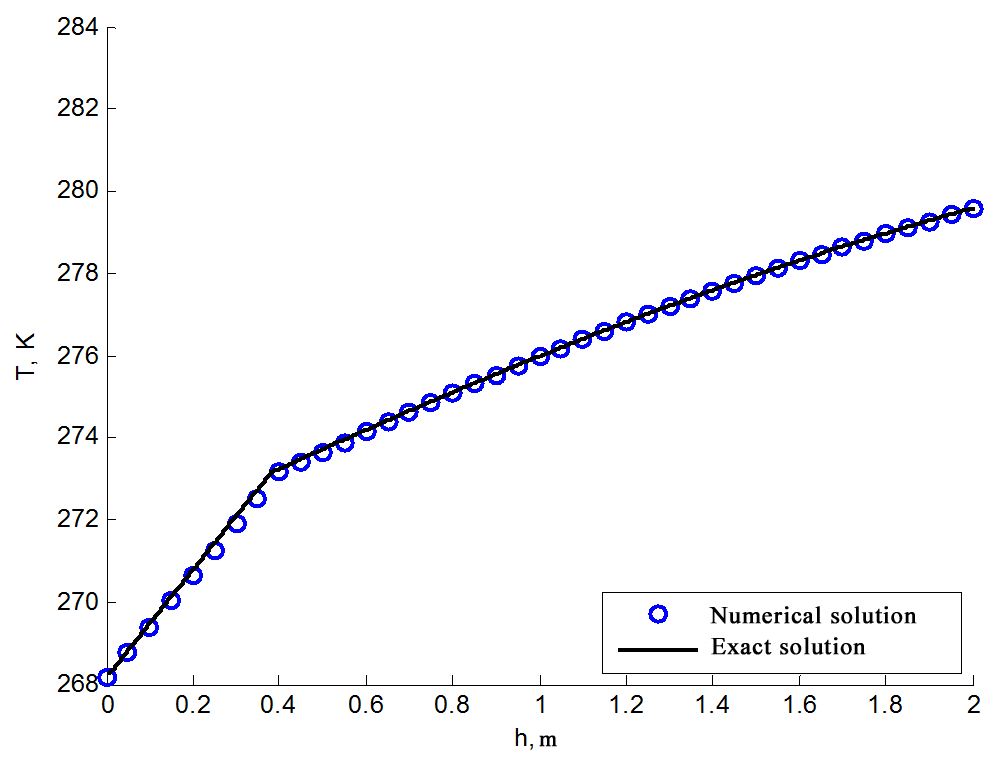Figure 4: Results of 4th numerical experiment

### Frost 3D verification against experimental data

The freezing of a cylindrical sample of peat with a moisture content of wtot=5 kg/kg and density ρd=160 kg/m3 was analyzed . The following thermophysical properties are applied in compliance with SNIP 2.02.04-88 Norms “Bases and Foundations on Permafrost”: volumetric heat capacity of thawed (Cth) and frozen (Cf) states, heat conductivity of thawed (λth) and frozen (λf) states (Table 2).

Table 2: Thermophysical Properties of Ground

 λth, W/(m×°С) λf, W/(m×°С) Cth, kJ/(m3×°С) Cf , kJ/(m3×°С) 0.41 0.70 3320 2100

The dependence of the unfrozen water content on temperature is given for peat in compliance with SP 25.13330.2012 Norms (SNiP 2.02.04-88 Norms “Bases and Foundations on Permafrost”). From the expression (B.5), the dependence takes the form shown in the Figure 5.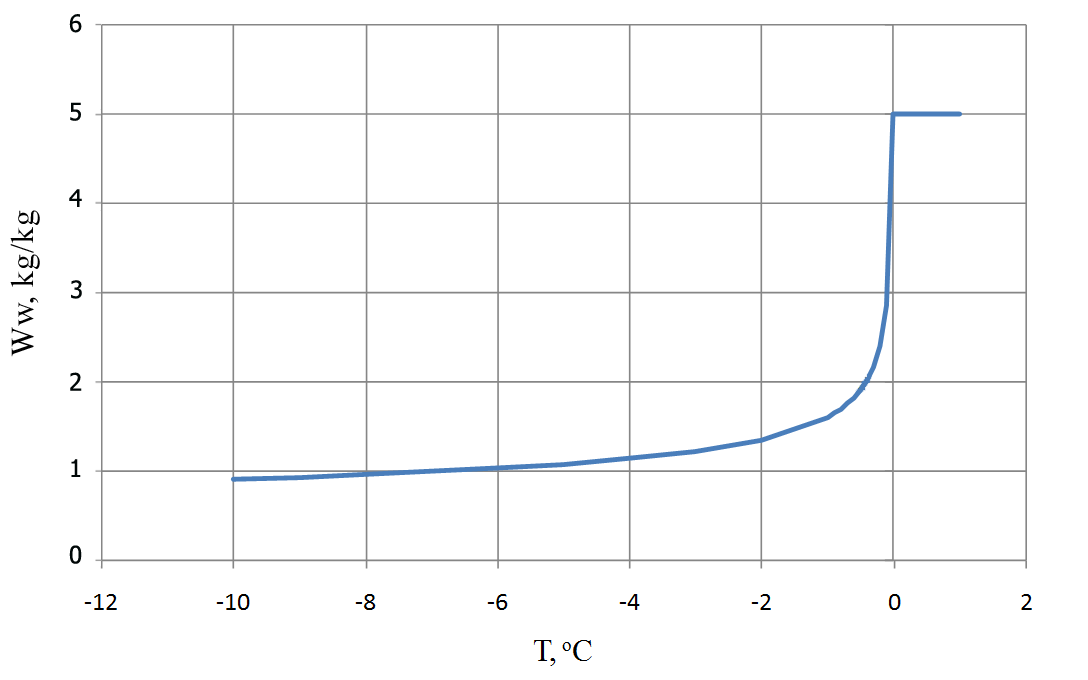Figure 5: Dependence of unfrozen water content on the temperature

The initial temperature of the peat sample is 283 K. Using directional cooling unit, the temperature at the end of the cylindrical sample is maintained at 268 K. The experimental temperature distribution data resulting from the freezing of the sample is presented in Figure 6 . Note that this laboratory experiment is comparable to the natural processes of ground freezing.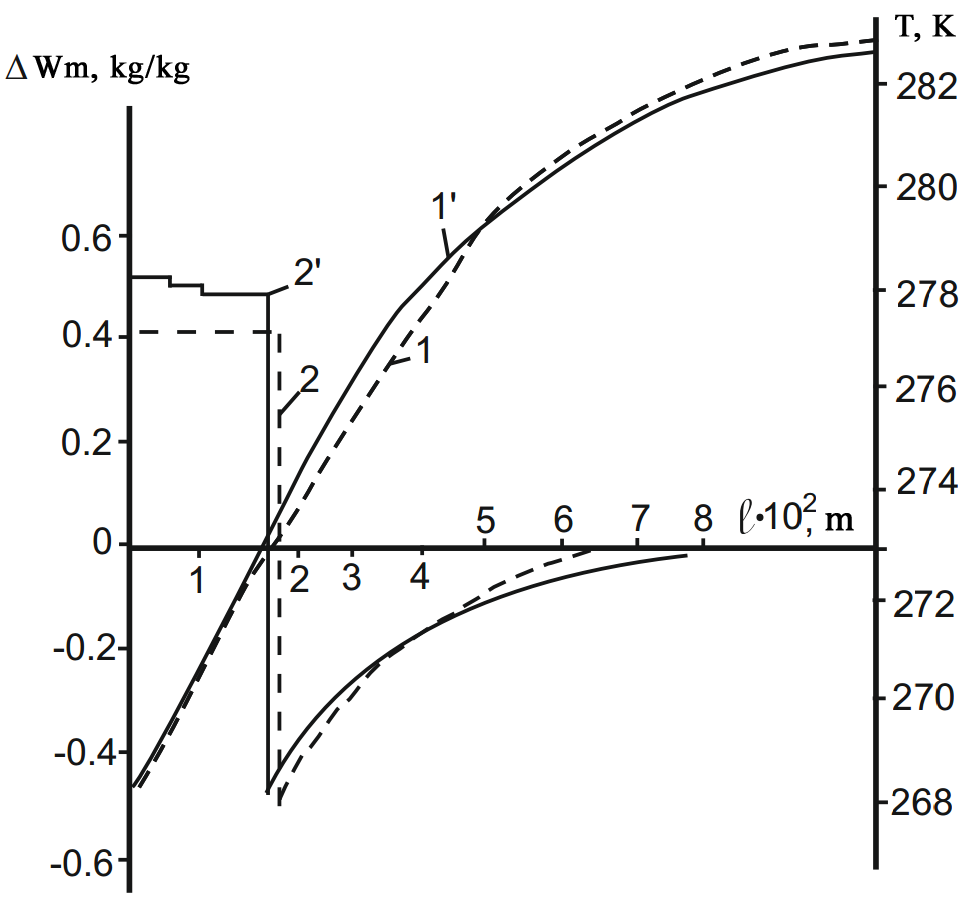Figure 6: Computational (1, 2) and experimental (1’, 2’ ) temperature distribution and moisture content

after freezing: W=5 kg/kg, ρ=160 kg/m3, T0=283 K, Tc=268 K, t=2.0 h 

The computational experiment for the same problem was conducted in a Frost 3D. The computed temperature distribution was compared with the experimental data (Figure 7), and the discrepancy between the two for the depth of frost penetration was 5%: a 0.020 m computational value for frost depth against the 0.019 m experimental measurement of the same. Note that the main contribution to the total error is the inaccurate determination of the thermophysical properties of the ground.Figure 7: Comparison of computational and experimental data

REFERENCES

1. Kislitsin A.A., Shabarov A.B. Heat and Mass Transfer. – Tyumen: TGU, 2007.

2. Brovka G.P. Interrelated Processes of Heat and Mass Transfer in Dispersive Media. – Minsk: Belarus. Nauka, 2011.